NESS ENGINEERING
TECHNICAL DATA
SURFACES AND VOLUMES OF COMMON SHAPES
Cylinder  Sphere Hollow Cylinder  Cone Torus Spherical Sector  Cylinder Portion

Cylinder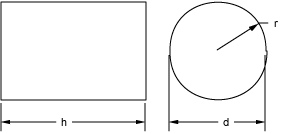Equations Configuration Where:Cylinder Surface r is the radius  d is the diameter  h is the height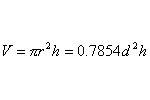Cylinder Volume r is the radius  d is the diameter  h is the height

Sphere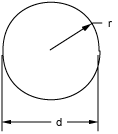Equations Configuration Where:Sphere Surface r is the radius  d is the diameter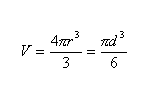Sphere Volume r is the radius  d is the diameter

Hollow Cylinder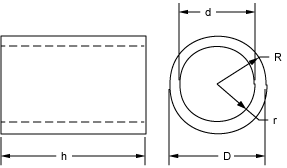Equations Configuration Where:Hollow Cylinder Volume R is the outer radius  r is the inner radius  D is the outer diameter  d is the inner diameter  h is the height  t is the thickness

Cone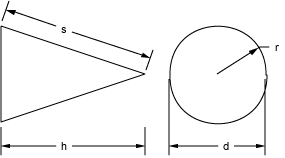Equations Configuration Where: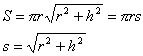Cone Surface r is the base radius  h is the heightCone Volume r is the base radius  d is the base diameter  h is the height

Torus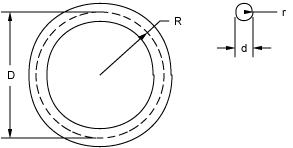Equations Configuration Where:Torus Surface R is the mean radius  r is the minor radius  D is the mean diameter  d is the minor diameter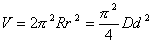Torus Volume R is the mean radius  r is the minor radius  D is the mean diameter  d is the minor diameter

Spherical Sector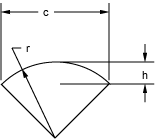Equations Configuration Where:Spherical Sector Surface r is the radius  h is the height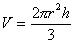Spherical Sector Volume r is the radius  h is the height

Cylinder PortionEquations Configuration Where: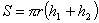Cylinder Portion Surface r is the radius  h1 is the 1st height  h2 is the 2nd heightCylinder Portion Volume r is the radius  h1 is the 1st height  h2 is the 2nd height

##### Send consulting inquiries, comments, and suggestions to nessengr@san.rr.com

Ness Engineering, Inc.
P.O. Box 261501
San Diego, CA 92196
(858) 566-2372
(858) 240-2299 FAX

 © Richard M. Ness and Ness Engineering, Inc. Last Updated: